# BALANCING CHEMICAL REACTIONS Chapter 6 CHEMICAL REACTIONS Signal

• Slides: 16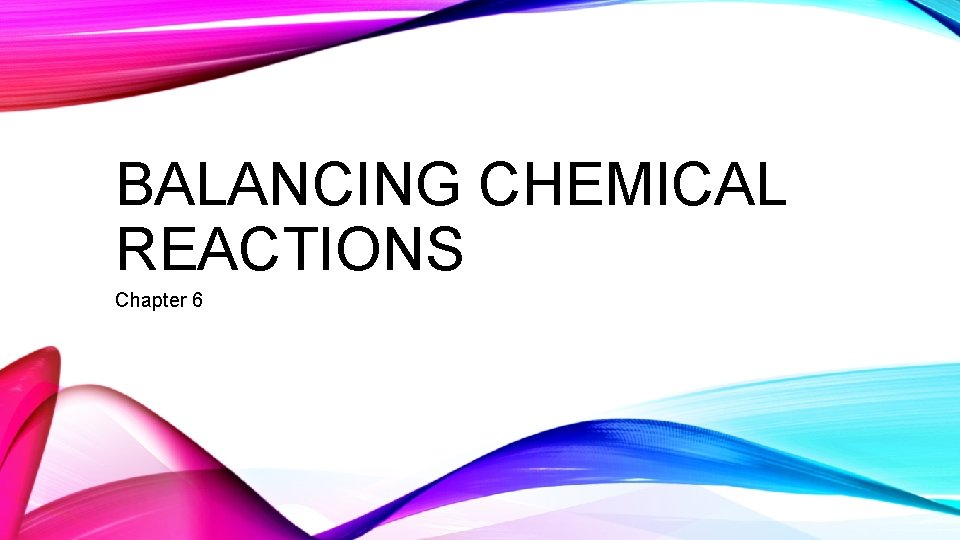BALANCING CHEMICAL REACTIONS Chapter 6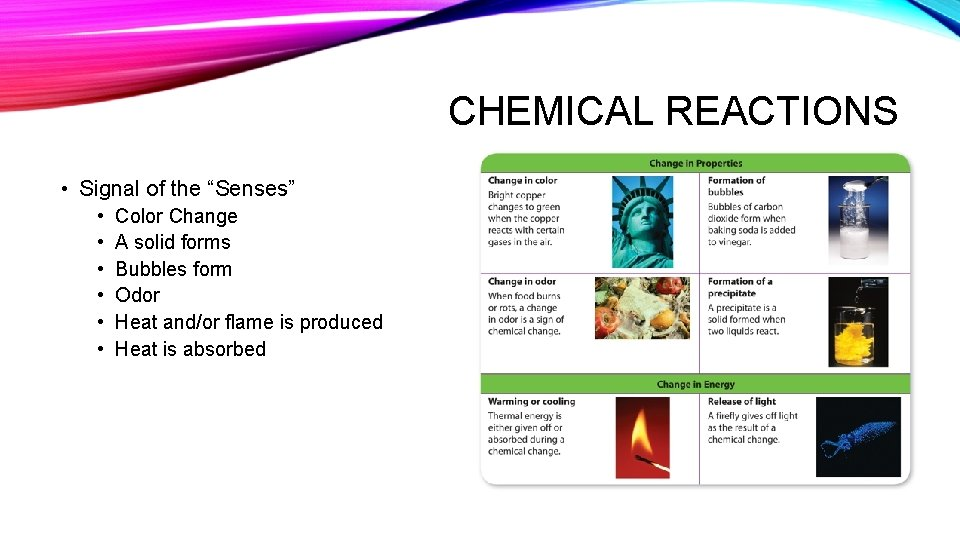CHEMICAL REACTIONS • Signal of the “Senses” • • • Color Change A solid forms Bubbles form Odor Heat and/or flame is produced Heat is absorbed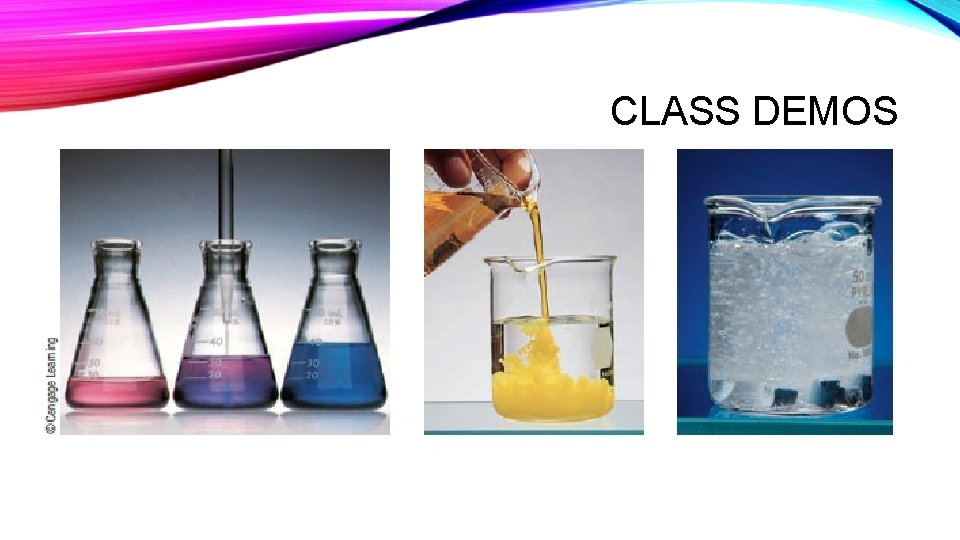CLASS DEMOS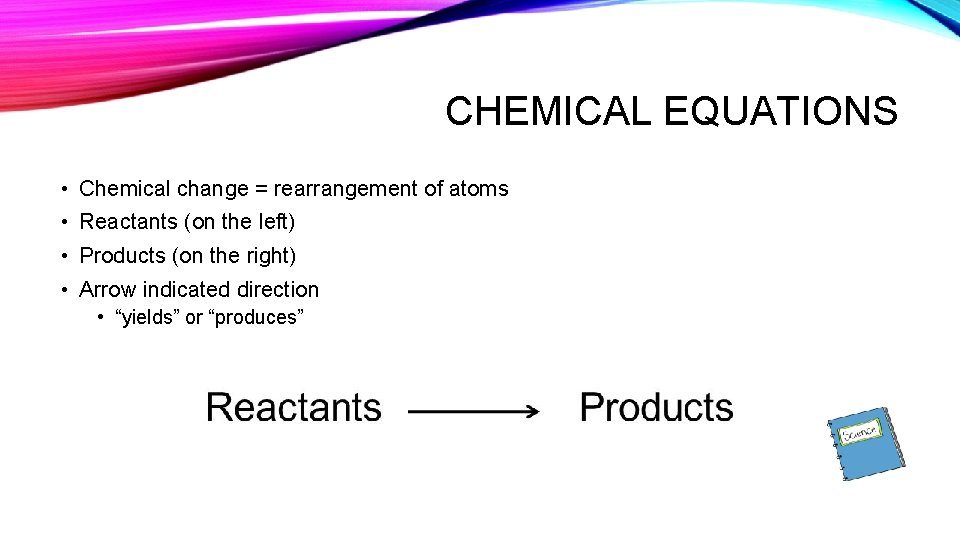CHEMICAL EQUATIONS • Chemical change = rearrangement of atoms • Reactants (on the left) • Products (on the right) • Arrow indicated direction • “yields” or “produces”WRITING CHEMICAL REACTIONS • Group like molecules together • Use coefficient to designate more than one • Designate physical state • • (s) (l) (g) (aq) solid liquid gas aqueous solution (dissolved in water)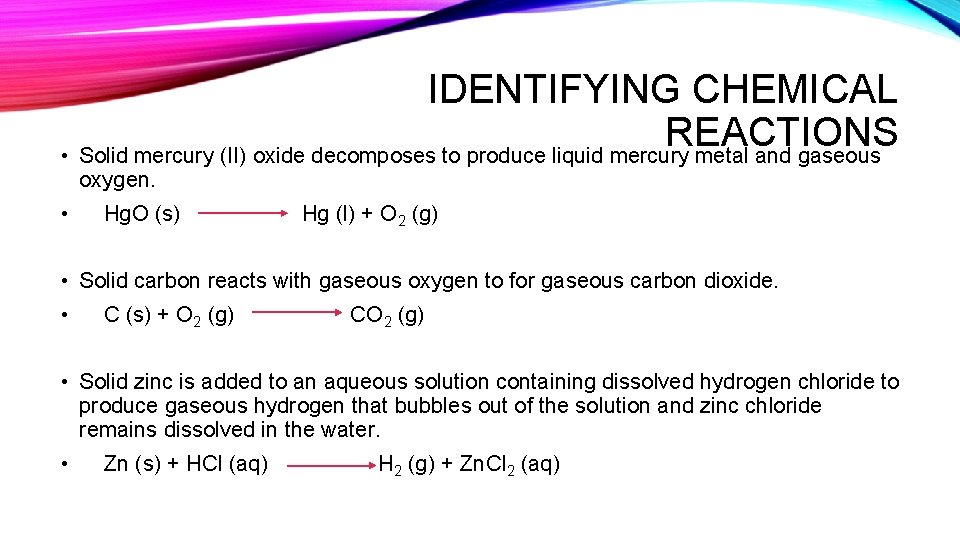IDENTIFYING CHEMICAL REACTIONS • Solid mercury (II) oxide decomposes to produce liquid mercury metal and gaseous oxygen. • Hg. O (s) Hg (l) + O 2 (g) • Solid carbon reacts with gaseous oxygen to for gaseous carbon dioxide. • C (s) + O 2 (g) CO 2 (g) • Solid zinc is added to an aqueous solution containing dissolved hydrogen chloride to produce gaseous hydrogen that bubbles out of the solution and zinc chloride remains dissolved in the water. • Zn (s) + HCl (aq) H 2 (g) + Zn. Cl 2 (aq)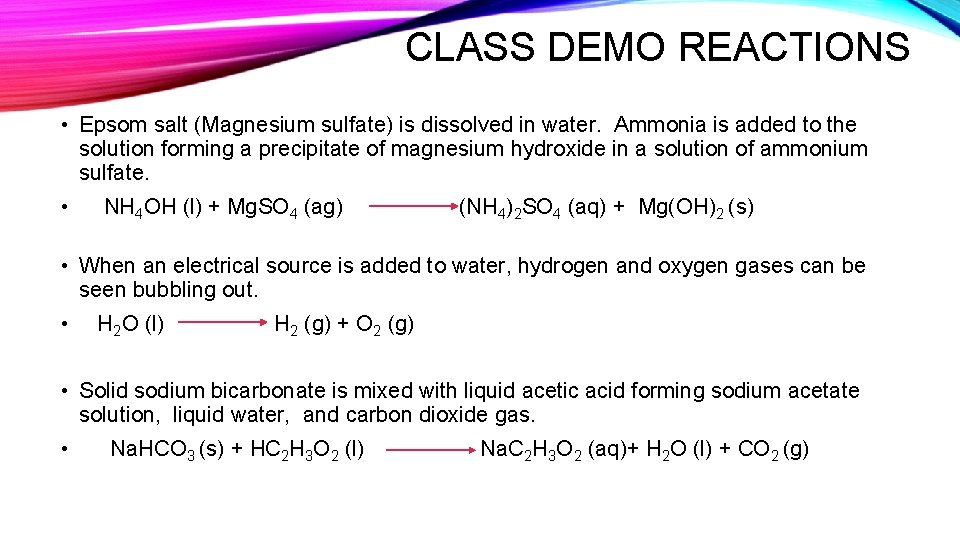CLASS DEMO REACTIONS • Epsom salt (Magnesium sulfate) is dissolved in water. Ammonia is added to the solution forming a precipitate of magnesium hydroxide in a solution of ammonium sulfate. • NH 4 OH (l) + Mg. SO 4 (ag) (NH 4)2 SO 4 (aq) + Mg(OH)2 (s) • When an electrical source is added to water, hydrogen and oxygen gases can be seen bubbling out. • H 2 O (l) H 2 (g) + O 2 (g) • Solid sodium bicarbonate is mixed with liquid acetic acid forming sodium acetate solution, liquid water, and carbon dioxide gas. • Na. HCO 3 (s) + HC 2 H 3 O 2 (l) Na. C 2 H 3 O 2 (aq)+ H 2 O (l) + CO 2 (g)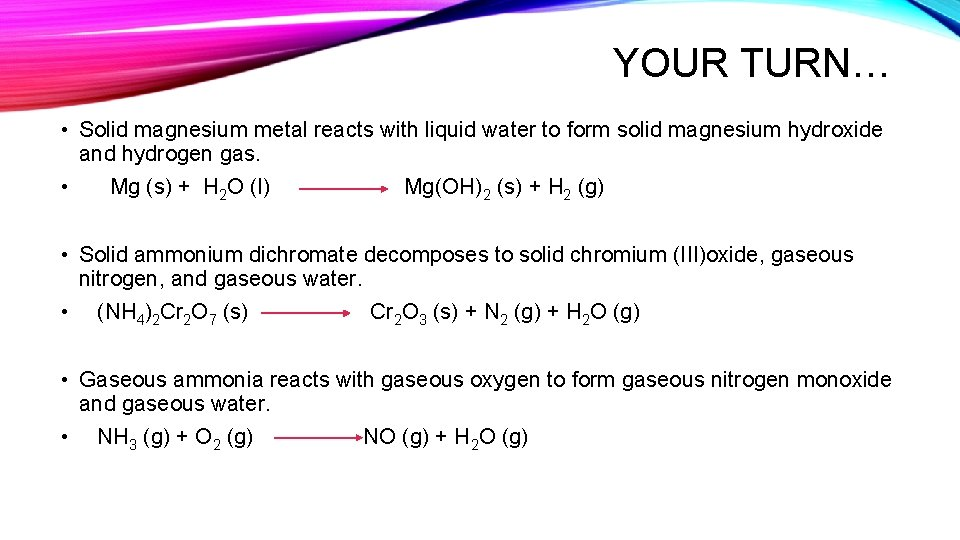YOUR TURN… • Solid magnesium metal reacts with liquid water to form solid magnesium hydroxide and hydrogen gas. • Mg (s) + H 2 O (l) Mg(OH)2 (s) + H 2 (g) • Solid ammonium dichromate decomposes to solid chromium (III)oxide, gaseous nitrogen, and gaseous water. • (NH 4)2 Cr 2 O 7 (s) Cr 2 O 3 (s) + N 2 (g) + H 2 O (g) • Gaseous ammonia reacts with gaseous oxygen to form gaseous nitrogen monoxide and gaseous water. • NH 3 (g) + O 2 (g) NO (g) + H 2 O (g)BALANCING CHEMICAL EQUATIONS • Products contain same number of atoms as reactants • Atoms cannot be created or destroyed ONLY rearranged • Same number of each type of atom on both sides of the arrow • Formulas of the compounds are NEVER changed in balancing the equation. Carbon – 1 Hydrogen – 4 Oxygen – 4HOW TO WRITE AND BALANCE EQUATIONS • 1. Determine the reactants, the products, and their states. • 2. Write the appropriate formula. • 3. Write an unbalanced equation. • 4. Start with most complicated molecule, start balancing the equation by adding the necessary coefficients so that the same number of atoms appears on both sides of the equation. • 5. Continue with all the molecules in the formula. • 6. Double-check to see that the same number of atoms are on both sides of the equation. • 7. Make sure coefficients are smallest number possible.BALANCING EQUATIONS • Hydrogen gas reacts with oxygen gas to produce liquid water • H 2 (g) + O 2(g) = H 2 O (l) • 2 H 2 (g) + O 2(g) = 2 H 2 O (l) Liquid ethanol reacts with oxygen gas to produce gaseous carbon dioxide and water vapor. • C 2 H 5 OH (l) + O 2 (g) = CO 2 (g) + H 2 O(g) • C 2 H 5 OH (l) +3 O 2 (g) = 2 CO 2 (g)+ 3 H 2 O (g) • Solid iron reacts with oxygen gas to for iron (III) oxide • Fe(s) + O 2 (g) = Fe 2 O 3 (s) • 4 Fe(s) + 3 O 2 (g) = 2 Fe 2 O 3 (s)MORE BALANCING EQUATIONS • Diboron trioxide reacts with liquid water to form boric acid. • B 2 O 3 (s) + H 2 O (l) = H 3 BO 3 (aq) • B 2 O 3 (s) + 3 H 2 O (l) = 2 B(OH)3 (aq) • Solid potassium reacts with liquid water to form gaseous hydrogen and potassium hydroxide dissolved in water. • K (s) + H 2 O (l) = H 2 (g) + KOH (aq) • 2 K (s) + 2 H 2 O (l) = H 2 (g) + 2 KOH (aq) • Ammonia gas reacts with oxygen gas to produce gaseous nitrogen monoxide and water vapor. • NH 3 (g) + O 2(g) = NO (g) + H 2 O(g) • 4 NH 3 (g) + 5 O 2(g) = 4 NO (g) + 6 H 2 O(g)WORKING WITH A PARTNER • One partner get out a computer, the other get some scrap paper. • On the pink card you were given, write down the names of both partners. • Go to this website… http: //www. sciencegeek. net/APchemistry/APtaters/Equation. Balancing. htm • Work all 20 problems then click the “Check” Button in the bottom center. • Write your score on the pink card. • Correct your mistakes and click “Check” again. • Write your corrected score on your pink card and turn in.MORE EQUATIONS TO BALANCE • Propane gas (used for grills) leaved the tank and vaporizes to mix with oxygen to form carbon dioxide gas and water as it burns. • C 3 H 6 (g) + O 2 (g) = CO 2 (g) + H 2 O(g) • C 3 H 6 (g) + 5 O 2 (g) = 3 CO 2 (g) + 4 H 2 O(g) • Hydrofluoric acid solution reacts with the silicone dioxide in glass to form a gaseous silicon tetrafluoride and liquid water. • HF (aq) + Si. O 2 (s) = Si. F 4 (g) + H 2 O (l) • 4 HF (aq) + Si. O 2 (s) = Si. F 4 (g) + 2 H 2 O (l)AND MORE EQUATIONS TO BALANCE • Solid ammonium nitrite is heated, it produces nitrogen gas and water vapor. • NH 4 NO 2 (s) = N 2 (g) + H 2 O (g) • NH 4 NO 2 (s) = N 2 (g) + 2 H 2 O (g) • Gaseous nitrogen monoxide produces dinitrogen monoxide gas and nitrogen dioxide gas. • NO (g) = N 2 O (g) + NO 2 (g) • 3 NO (g) = N 2 O (g) + NO 2 (g) • Liquid nitric acid decomposes to reddish brown nitrogen dioxide gas, liquid water and oxygen gas. • HNO 3 (l) = NO 2 (g) + H 2 O (l) + O 2 (g) • 4 HNO 3 (l) = 4 NO 2 (g) + 2 H 2 O (l) + O 2 (g)WORK CITED • https: //www. cinchlearning. com/clarity/cinch/glencoe_science_2012_texas/images/e books/sci 8/334_1/sci_334_1_figure 1. jpg • http: //chemistrysaanguyen. weebly. com/uploads/1/3/3/1/13315249/9024879_orig. pn g? 1 • http: //images. slideplayer. com/27/9187628/slides/slide_2. jpg • http: //blogs. fairview. k 12. oh. us/ginhou 215/files/2016/05/Equations-28 qlez 3. png • https: //www. learner. org/courses/chemistry/images/lrg_img/methane_rxn. jpg • https: //archives. library. illinois. edu/erec/University%20 Archives/1505050/Rogers/Tex t 3/Tx 34/tx 34 p 1. GIF